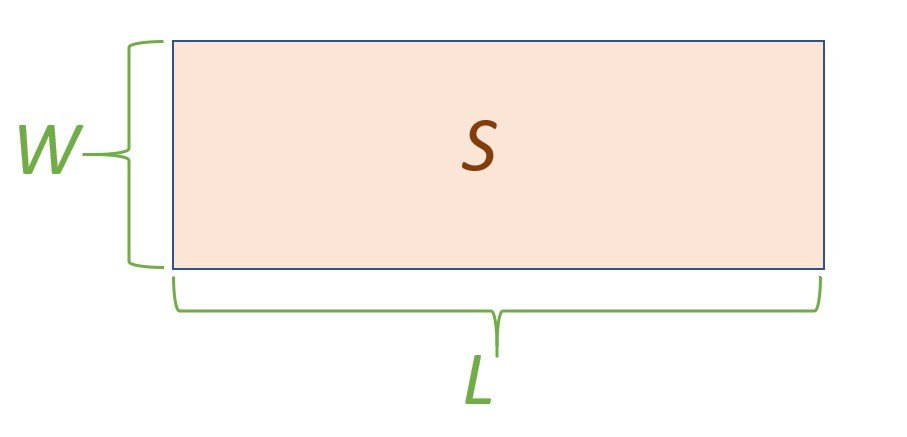# Square meters to Linear meters – sqm to lm – m2 to lin. m

Enter the area in m2 (square meters):

Enter the width of the linear meter in m (meters):

The result in linear meters (lin.m, lm) is:

0.00lm

## Calculator reference

The figure below shows the material measured in linear meters:Legend: L – length in linear meters, W – width of a linear meter, S – area.

## How to convert a square meter to a linear meter?

You need to divide the area in square meters by the width of a linear meter.

A linear meter is determined by the formula:

L = S / W

L is the length in linear meters (lin. m, lm);

S is the area in square meters (sqm, m2);

W is the width of a linear meter (m).

Figure with legend is shown above.

### For example:

Find the length of the carpet in linear meters. The area of ​​the carpet is 15 m2, the width of the carpet is 3 m.

L = S / W = 15/3 = 5 lm